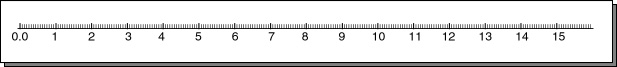`28 = 256`

# Limited Precision

This is an easy question. If `N` bits are used, then only `2N` things can be represented, no matter what type of things they are. Eight bits can represent 256 positive integers (unsigned binary), 256 positive and negative integers (two's complement), 256 fractional numbers (our fixed point method), and so on. Here is a number line showing the 256 values that can be represented with this fixed notation:The smallest value is zero (`00000000`). The largest value is 15.9375 (`11111111`). The smallest non-zero value is 0.0625. Every represented value is a multiple of 0.0625.

Another way to think of fixed point notation is that the eight bits represent an integer from 0 to 255. The integer is a multiplier of the value 0.0625.

The range between any two integers is divided into 16 numbers (because 16*0.0625 = 1.0). This is not very many divisions. There are big gaps between represented numbers.

Important points: With our version of 8-bit fixed point, the only numbers that can be represented lie in the range zero to 15.9375. This is called a limit in range. Also, only a few real numbers within that range can be represented. This is called a limit in precision.

All schemes for representing real numbers with bit patterns have limited range and limited precision. This is true for the usual floating point representations of computers. No matter how many bits are used, there are limits on the range and precision.

### QUESTION 10:

(Calculus Review: ) How many real numbers lie between 0.0 and 0.0625?